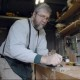## A Question of Proportion

Ok, why does one shape “work” and another not? This has been a topic of research for I don’t know how long.

Great art seems to conform to the proportions laid out in the golden section or golden rectangle. Getting to that relationship is easy, using story sticks and some tricks based upon math. You don’t need extensive mathematical knowledge. I, however, had to run the gamut of algebra, geometry, and trigonometry before the simplicity of this ratio got through. You can skip the MATH section, and go to STORY STICKS if you don’t want to know all that part.

MATH

The golden section deals with the relationship of the sides of a rectangle that seems most pleasing to the human eye and sense of proportion. The relationship is stated as “the width is to the length as the length is to the width-plus-length. As an algebraic expression it would be W/L=L/(W+L). You can manipulate this expression to arrive at W2+LW−L2 = 0, where L = length and W = width. In geometric terms, this says that the area of a square L by L is equal to the combined areas of a square W by W and a rectangle L by W. After playing around with the algebraic expression W2+LW−L2=0, I allowed L=2 and solved for W. Thus if L=2, W=√(5)−1. We will return to those numbers in a moment. But First we need to check on the geometry.
• Area of the square L by L is 2 * 2 = 4
• Area of the square W by W is (√(5)−1) * (√(5)−1) = 6−(2*√(5))
• Area of the rectangle L by W is 2 * (√(5)−1) = (2*√(5))−2

So, 6−(2*√(5)) + (2*√(5))−2 equals 4 which is the same as the area of the large square, L by L.

Geometry agrees with algebra.

What about trigonometry, that study of three sided figures? Can it help us?

Return to the numbers we derived from algebra: L=2, W=√(5)−1. After some time, it dawned on me that these three numbers form the sides of an easily constructed, right triangle, ABC.

Start with line segment AB. Construct perpendiculars at A and B. Bisect segment AB at G. With the point of your compass on A, swing an arc from G to establish point C. Draw line segment BC. This right triangle now has side AC equal to one unit, side AB equal to two units, and side BC equal to √(5) units. These are the three numbers we arrived at in our algebra.

With the point of your compass on C, swing an arc from A to establish D. Line segment BD equals √(5)−1 units. Thus if the length equals segment AB, the width of the golden rectangle equals segment BD. With your compass on point B, swing an arc from D to establish point F. With the compass on point A, use the same radius to establish point E. Thus rectangle ABFE is a golden rectangle. And we have harmony between three branches of mathematics at least.

STORY STICKS

The use of this information lies in making story sticks rather than in making calculations or constructions. Cut three sticks: L, B, and C. Stick L is the length of your proposed project. Cut stick B to one half the length of stick L. Lay these two to form a right angle (use a framing square to establish the right angle) and cut stick C to form the hypotenuse. Shorten stick C by the length of stick B to form stick W. Thus L is your length and W is the width to make your project conform to the proportions of the golden section.

COMING

Next post I will discuss how the golden section can apply to woodturning where we are dealing with varying degrees of round rather than rectangular.

Ellis HeinI am a woodturner and the author of The Woodturner's Project Book. I have a life-long interest in the gospel preached by George Fox and the early Quakers. You can see some of my material on that subject at http://nffquaker.org/profiles/blog/list?user=1zw2th7nj9p89.
This entry was posted in Art and tagged , , , . Bookmark the permalink.

### 2 Responses to A Question of Proportion

1.yaakov says:

I have never acutally seen the math behind this, and to be honest, I never really gave it a second thought, which I find somewhat suprising because I am generally a very curious fellow who likes to know how things work. I find math to be a beautiful art, I like the symmetry of it. Even more interesting is how your brain and eye can just see that something is “right.”

Last year I completed a very special chest of drawers, and normally I would use the “golden ratio” to make it look “right”, but the piece used special numerical deminsions. I’m not going to say the piece does not “Look right”, but it does not my designing eye is unsettled when I look at it. Just goes to show, that when HaShem created the universe He saw made beauty in math too.

Looking forward to the next installment.

Kol tuv,
yaakov….

•Ellis Hein says:

Thanks for your comment. I may have created a level of expectation regarding the next installment that I don’t live up to. It is nice to create an interesting post, and I always hope that I can keep the trend going up.